# 热乎着，昨晚阿里这题真太绝了

joytrian

0收藏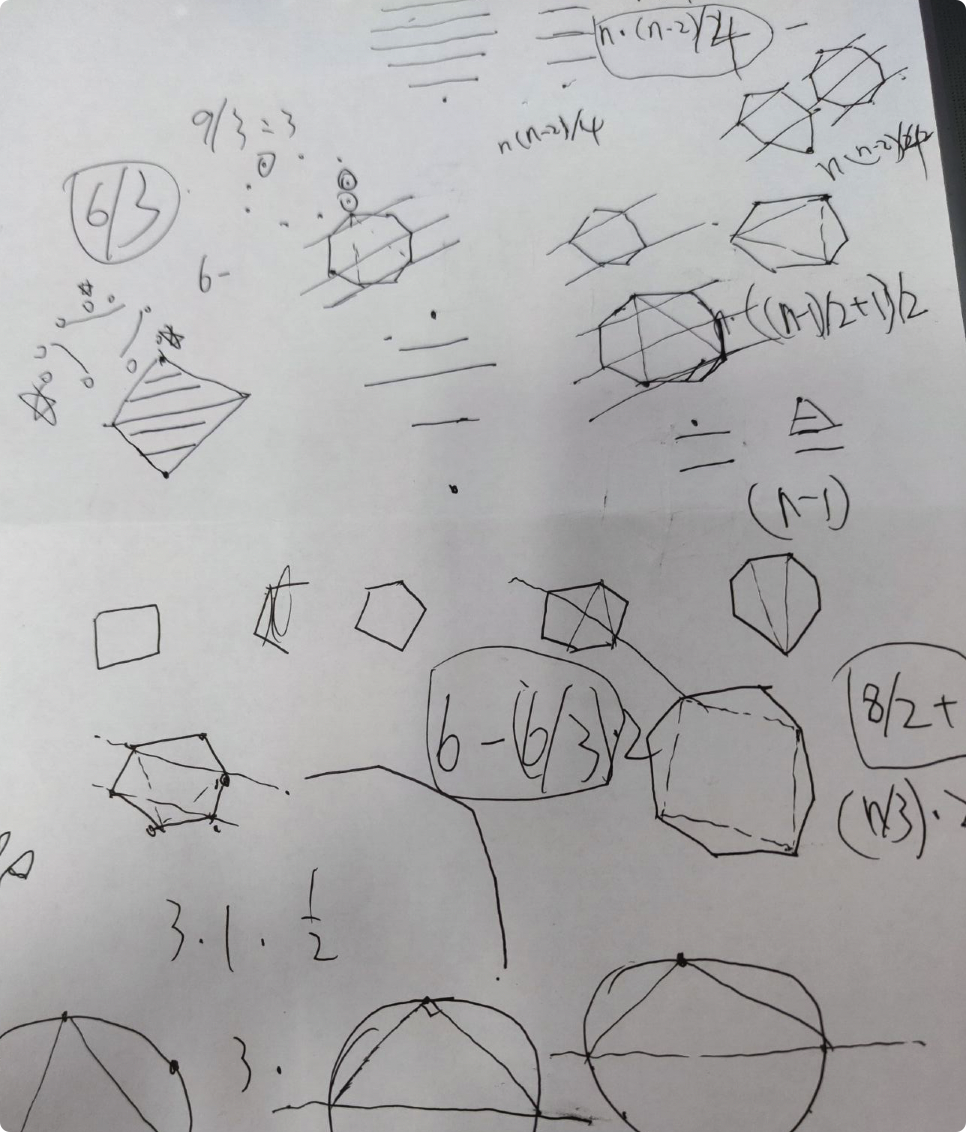瞎折腾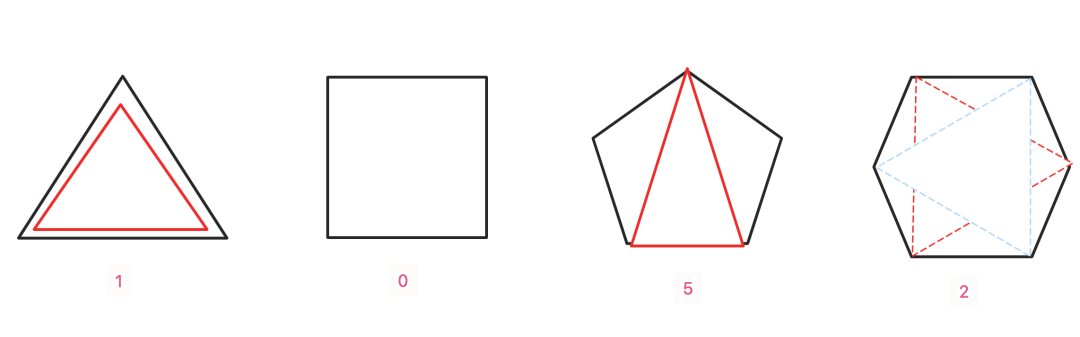• 正多边形，多少边就是多少个顶点
• 正多边形，有轴对称的特性，等腰锐角三角形，也有轴对称的特性。
• 等腰锐角三角形，腰有两个，而底边有一个，要么从底边考虑，要么从顶点考虑，这里底边如上面的正6边型甚至更多边型显然不容易考虑，但是各个顶点都是多边形的顶点，所以肯定从顶点考虑。算出一个顶点为等腰锐角顶角的所有三角形乘以顶点数量(如正五边形)然后减去重复(如正3、正6边形)的就是总结果了。
• 奇数偶数分开讨论。
偶数情况
我们先用偶数的情况分析，先不考虑重复情况(考虑太多脑子混淆)，将 图形摆一下成这样：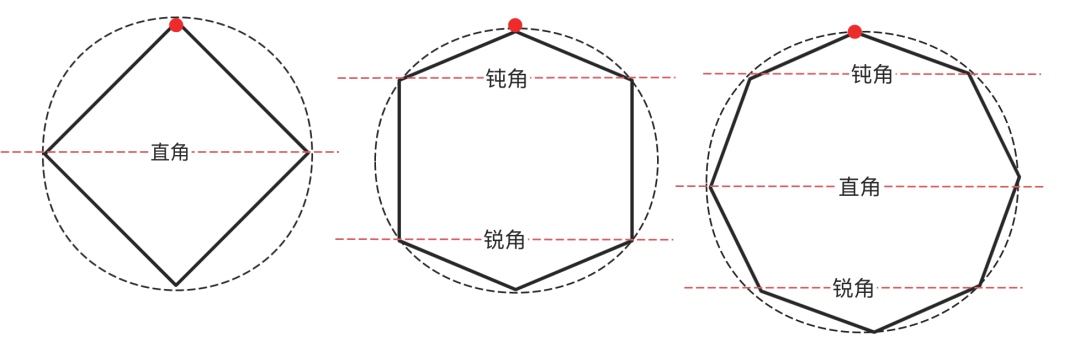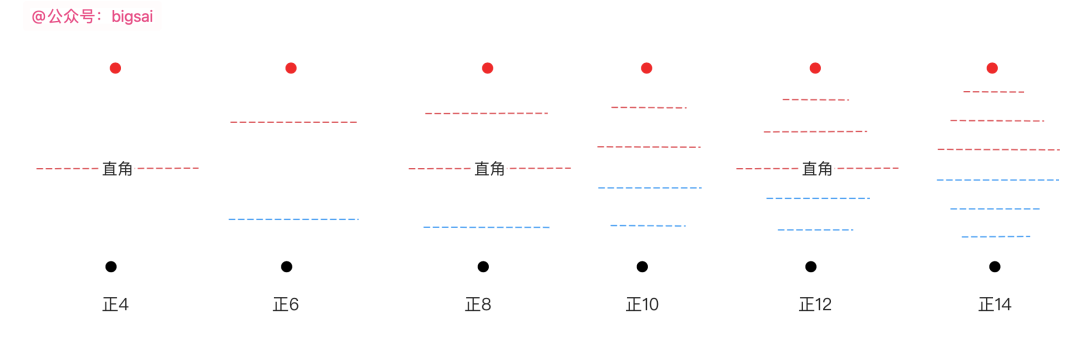``total=n*((n-2)/4))``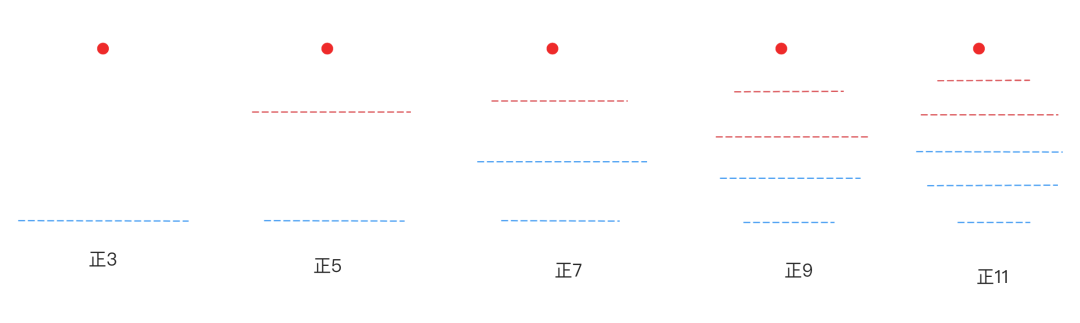``total=n*(((n-1)/2+1)/2))``

`` total-=(n/3)*2;``

``````public static void main(String[] args) {
Scanner sc=new Scanner(System.in);
int n=sc.nextInt();

long total;
if(n%2==0)
total=n*((n-2)/4);
else
total=n*(((n-1)/2+1)/2);
if(n%3==0){
total-=(n/3)*2;
}
System.out.println(total);
}``````

``````total=n*((n-2)/4);
total=n*(((n-1)/2+1)/2);``````

``````int temp=n*((n-2)/4);
long total=temp;``````

`````` double a=3/2;
System.out.println(a);``````

`` double a=(double) 3/2;``

``````public static void main(String[] args) {
Scanner sc=new Scanner(System.in);
int n=sc.nextInt();
long total;
if(n%2==0)
total=(long)n*((n-2)/4);
else
total=(long)n* (((n-1)/2+1)/2);
if(n%3==0){
total-=(n/3)*2;
}
System.out.println(total);
}``````